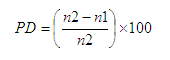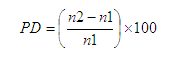﻿ Percent Difference
You are here: Processor Library > Matching > Comparisons > Percent Difference

# Comparison: Percent Difference

The Percent Difference comparison calculates the percentage difference between two number values in order to determine how close they are, relative to the larger value.

The Percent Difference (PD) between two numbers is calculated in one of two ways as follows:

If Use larger number as denominator (see Options below) = Yes:of, if Use larger number as denominator = No:where:

PD = Percent Difference

n1 = the smaller of the two numbers being compared

n2 = the larger of the two numbers being compared

So, for the pair of values "50" and "75":

n1 = 50, and

n2 = 75,

resulting in a Percent Difference of 75-50 = 25/75 = 0.33 *100 = 33% if Use larger number as denominator = Yes, or

75-50 = 25/50 = 0.50*100 = 50% if Use larger number as denominator = No

## Use

Use the Percent Difference comparison to match number values that are relatively close to one another. This is useful when comparing number values such as order amounts that may range from very low to very high, meaning an Absolute Difference comparison would lead to misleading results in terms of how similar two numbers are to each other. For example, the values "0.5" and "1.20" might be considered as much weaker matches than the values "8200" and "8300".

This comparison supports the use of result bands.

## Options

 Option Type Purpose Default Value Match No Data pairs? Yes/No This option determines the result of a comparison when it compares two No Data values for an identifier. If set to No, the comparison will give a 'no data' result when comparing a No Data value against another No Data value. If set to Yes, the comparison will give a full match (0% percent difference) when comparing a No Data value against another No Data value. A 'no data' result will only be returned if a No Data value is compared against a populated value. No Use larger number as denominator? Yes/No This option allows you to change the way the Percent Difference between two numbers is calculated as shown above. If set to Yes, the first equation above will be used, and the Absolute Difference between the two numbers will be related to the higher number. For example, "25" and "75" would have a Percent Difference of 67%. If set to No, the second equation above will be used, and the Absolute Difference between the two numbers will be related to the smaller number. For example, "25" and "75" would have a Percent Difference of 200%. Yes

## Example

In this example, the Percent Difference comparison is used to identify numbers that are relatively close to each other. The following options are specified:

Example configuration

Match No Data pairs? = No

Use larger number as denominator? = Yes

Example results

With the configuration above, the following table illustrates some example comparison results:

 Value A Value B Comparison result (Percent Difference) 50 75 33% 200 250 20% 0.005 0.0053 6% 4089 8044 49% Null Null no data

Oracle ® Enterprise Data Quality Help version 9.0